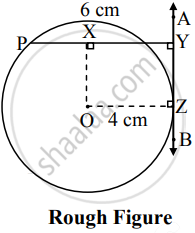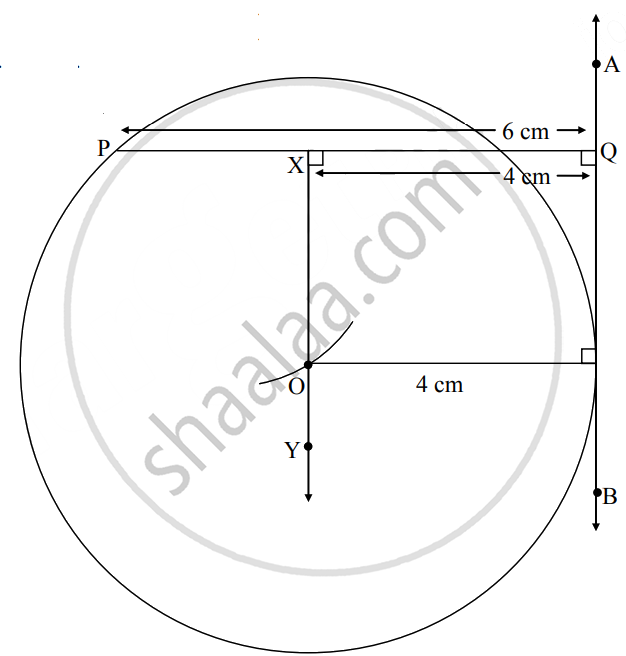# Point P is at a distance of 6 cm from line AB. Draw a circle of radius 4 cm passing through point P so that line AB is the tangent to the circle - Geometry

Diagram

Point P is at a distance of 6 cm from line AB. Draw a circle of radius 4 cm passing through point P so that line AB is the tangent to the circle

#### SolutionAnalysis: As shown in the figure, A circle of radius 4 cm has center O.

Perpendicular distance of P from line AB = 6 cm.

∴ PY = 6 cm and ∠PYA = 90°   ......(i)

seg OX is a perpendicular from point O to seg PY at point X.

Line AB is a tangent to the circle at point Z.

∴ OZ is radius of the circle.

In ▢XOZY,

∠X = 90°    ......[∵ OX ⊥ PY]

∠Y = 90°   ......[From (i)]

∠Z = 90°    ......[Tangent theorem]

∴ ∠O = 90°   ......[Remaning angle of ▢XOZY]

∴ ▢XOZY is a rectangle.

. ∴ seg ZY = seg OX and seg XO = seg YZ   ......[Opposite sides of a rectangle]

But OZ = 4 cm     ......…[Radius of the circle]

∴ XY = 4 cmSteps of construction:

1. Draw line AB.
2. From any point Q on line AB, draw a, perpendicular PQ of 6 cm.
3. Draw point X on seg PQ such that seg XQ = 4 cm.
4. Draw ray XY interior of the circle such that ∠QXY = 90°
5. Taking distance of 4 cm in compass, draw an arc with centre P on ray XY and name the point of intersection as O.
6. With centre O, draw a circle of radius 4 cm. Which is the required circle.
Concept: Basic Geometric Constructions
Is there an error in this question or solution?

Share